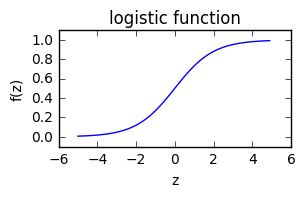Black-Box-Policy-Optimization slides

Here we demonstrate how the policy can be directly be learned by the cross entropy method (some kind of evolutionary algorithm) as suggested in the Open AI Deep Reinforcement Learning Tutorial.

The core idea of the cross entropy method is similar to evolution strategy[Wie14]:

• maintain a search distribution
• sampling from the search distribution
• and evaluate the samples for updating the search distribution, iteratively.

Here we use a search distribution a multivariate Gaussian with diagonal covariance matrix. To update the search distribution the best offsprings (elite) are used to calculate the new parameters of the Gaussian (the mean and the variance).

#### Cart Pole Problem¶

In :
import numpy as np
import numpy.testing as npt

import matplotlib.pyplot as plt
%matplotlib inline

In :
import gym
env = gym.make('CartPole-v0')

[2017-05-12 09:25:17,431] Making new env: CartPole-v0

In :
env.observation_space

Out:
Box(4,)
In :
observation = env.reset()
#[position of cart, velocity of cart, angle of pole, rotation rate of pole]
observation

Out:
array([-0.0258769 , -0.04715221,  0.01703425, -0.03715459])
In :
env.action_space

Out:
Discrete(2)

The action space is one-dimensional with two discrete actions $a \in \{0, 1\}$.

We use (similar to logistic regression) a log-linear model (logistic regression) for the probability of action $a=1$ for state $\vec s$ parametrized by $\theta = \{ b, \vec w\}$

$$p(a=1 \mid \vec s; \theta) = \frac{1}{1+\exp(-(\vec s \cdot \vec w)+b)}$$

The probability of action $a=0$ is $p(a=0 \mid \vec s; \theta) = 1-p(a=1 \mid \vec s; \theta)$

In :
def logistic_function(z):
return 1/(1 + np.exp(-z))

In :
plt.figure(figsize=(3, 1.5))
z = np.arange(-5, 5, 0.1)
plt.plot(z, logistic_function(z), 'b-')
plt.title("logistic function")
plt.xlabel('z')
plt.ylabel('f(z)')
plt.ylim(-0.1, 1.1)

Out:
(-0.1, 1.1)Sampling the action for an observation (state) and a fixed $\theta$ from $p(a \mid \vec s; \theta)$:

In :
def get_action(theta, observation):
p =  logistic_function(np.dot(theta[1:], observation) + theta)
return np.random.binomial(n=1, p=p, size=1)


Implementation of Algorithm 1: Cross Entropy Method:

Note: The environment stops automatically after 200 steps.

In :
def optimize_policy(nb_generations = 200,
nb_offsprings = 250,
nb_elite = 50,
n=5):

mu = np.random.rand(n) * 10. - 5.
cov = np.eye(n) * np.random.rand(n) * 10.

for ii in range(nb_generations):
# Draw random samples for the offsprings from a multivariate normal distribution
offsprings = np.random.multivariate_normal(mean=mu, cov = cov, size=nb_offsprings)
# the returns (fittness value) for each offspring
returns = np.zeros(nb_offsprings)
for i, theta in enumerate(offsprings):
done = False
observation  = env.reset()
return_ = 0
# run the agent in the environment to get the corresponding return (fitnesvalue)
while not done:
action = get_action(theta, observation)
observation, reward, done, info = env.step(action)
return_ += reward
returns[i] = return_

# sort the offsprings according to the fitness values
# and compute the new gaussian parameters (mu and cov) from the
# best offsprings
rang = np.argsort(returns)[::-1]
mu = offsprings[rang[:nb_elite]].mean(axis=0)
cov = np.eye(n) * offsprings[rang[:nb_elite]].var(axis=0)

#print progress
#if ii%10 == 0:
#    print (ii, returns[rang[:nb_elite]].mean())

if returns[rang[:nb_elite]].mean() >= 200.:
return mu

return mu

theta = optimize_policy()

In :
theta

Out:
array([ 0.15929439, -0.59793475,  1.06206644,  4.38717968,  3.77750642])

Use the following code snippet for

In :
observation  = env.reset()
for t in range(5000):
env.render()
action = get_action(theta, observation)
#print ("random action:", action)
observation, reward, done, info = env.step(action)
# observation =
#  [position of cart, velocity of cart, angle of pole, angular velocity of pole]
#print (observation, reward, done, info)
if done:
print("Episode finished after {} timesteps".format(t+1))
break

env.render(close=True)

Episode finished after 116 timesteps


#### Open Questions / Exercises¶

• Try other kinds of evolutionary algorithms, e.g. evolution stategy [Wie14] and compare the results.
• Does it help to fit the full covariance matrix not only the diagonal elements?